# Fundamental Particles of Matter Electrons Cathode Ray Tube

• Slides: 8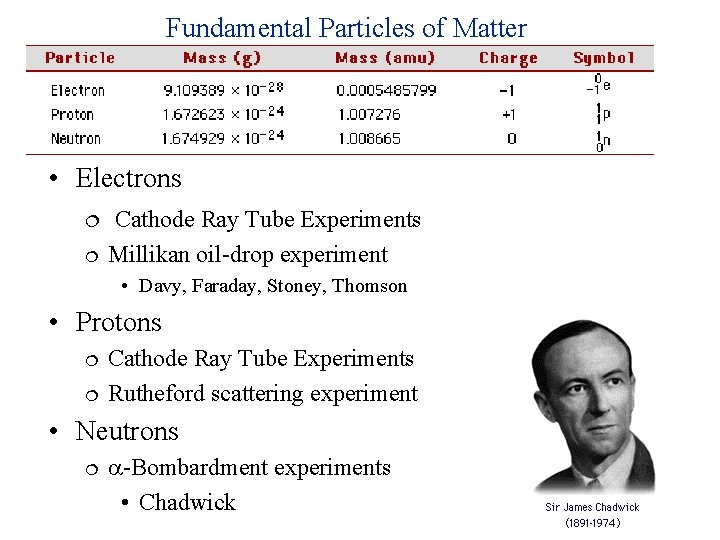Fundamental Particles of Matter • Electrons Cathode Ray Tube Experiments Millikan oil-drop experiment • Davy, Faraday, Stoney, Thomson • Protons Cathode Ray Tube Experiments Rutheford scattering experiment • Neutrons -Bombardment experiments • Chadwick• Cathode rays • Travel in straight lines • Negatively charged • Have mass • Cathode rays = Electron beam • Thomson determined the e/m ratio by studying the deflection of the beam in electric and magnetic fields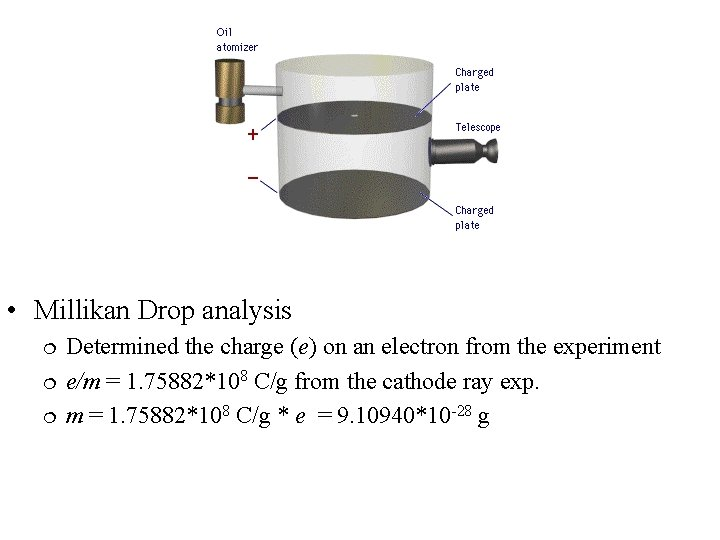• Millikan Drop analysis Determined the charge (e) on an electron from the experiment e/m = 1. 75882*108 C/g from the cathode ray exp. m = 1. 75882*108 C/g * e = 9. 10940*10 -28 gOriginal “Plum Pudding” Model • Protons • Cathode Ray Tube Experiments New Model Atom cation+ + e Canal Rays = Positive (cation) rays • H 2 2 H+ + 2 e • Determined the e/m of a proton • Rutheford Scattering Experiment Nucleus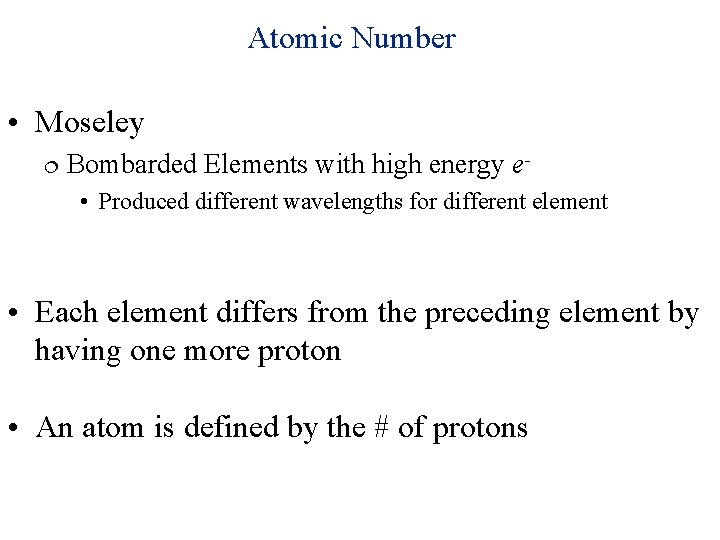Atomic Number • Moseley Bombarded Elements with high energy e • Produced different wavelengths for different element • Each element differs from the preceding element by having one more proton • An atom is defined by the # of protons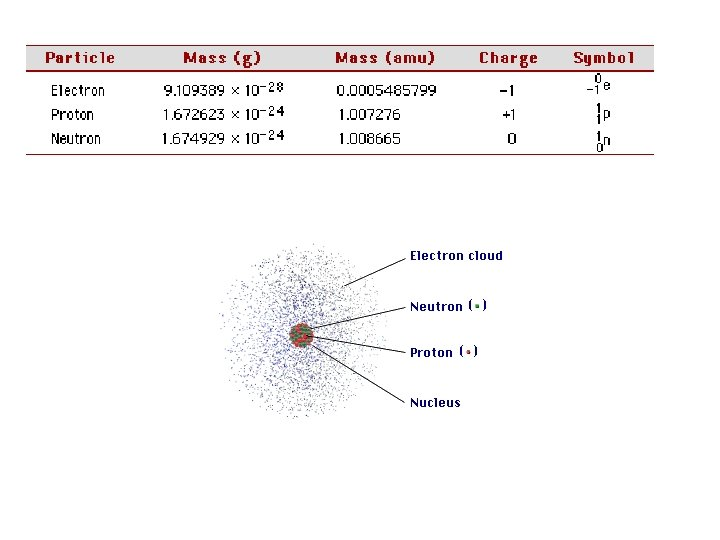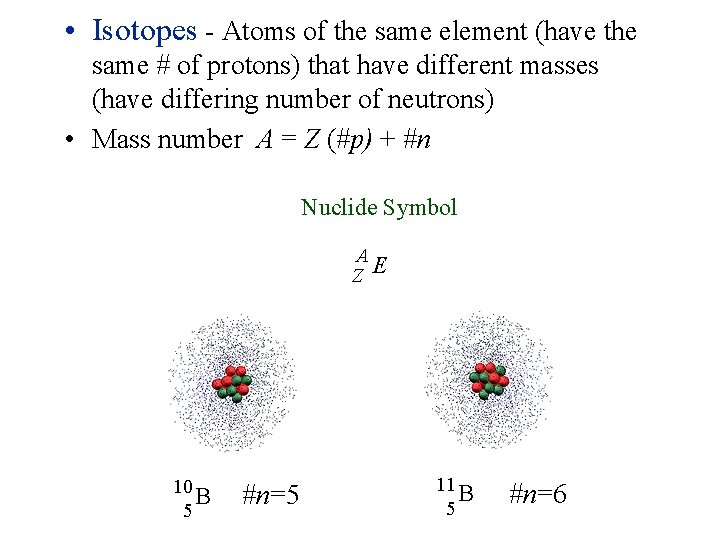• Isotopes - Atoms of the same element (have the same # of protons) that have different masses (have differing number of neutrons) • Mass number A = Z (#p) + #n Nuclide Symbol A Z E 10 B 5 #n=5 11 B 5 #n=6Mass Spectrometry - measures the charge-to-mass (e/m) ratio of charged particles. If we put Neon in a mass spectrometer we could determine how many natural isotopes there are for neon and the percent abundance of each isotope 20 Ne 21 Ne 22 Ne 90. 48% 0. 27% 9. 25% atomic mass of Ne = 19. 99244*0. 9048 + 20. 99384*0. 0027 + 20. 99384*0. 925 atomic mass Ne = 20. 1979 amu